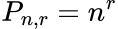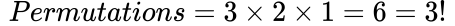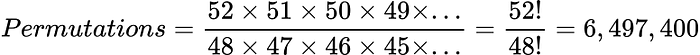# A Primer on Combinations and Permutations

Modelingposted by ODSC Community June 20, 2023

Combinations and permutations are common throughout mathematics and statistics, hence are a useful concept that we data scientists should know. In this post,...Combinations and permutations are common throughout mathematics and statistics, hence are a useful concept that we data scientists should know.

In this post, I want to discuss the difference between the two, the difference between the two, and also how one would calculate them for some given data.

## Overview and Simple Example

The main thing that differentiates between permutations and combinations is that for the former order does matter but it doesn’t for the latter.

For example, let’s say we have three different colored balls red, green, and blue and we want to put them in an arbitrary order such as:

```1: RED
2: GREEN
3: BLUE```

The combination of these three balls is 1 as each order will contain the same three combinations of ballsHowever, there are 6 permutations as we can have:

```1: RED    1: RED    1: GREEN  1: GREEN  1: BLUE.  1: BLUE
2: GREEN  2: BLUE   2: RED.   2: BLUE   2: RED    2: GREEN
3: BLUE   3: GREEN  3: BLUE.  3: RED    3: GREEN  3: RED```

Now you have a basic understanding of what combinations and permutations mean, let’s get more into the theoretical details!

## Permutations

Like we said, for permutations order is important and we want all the possible ways/lists of ordering something.

There are actually two types of permutations:

Let’s run through both!

## With Repetition

This one is pretty intuitive to explain. For example, given a padlock which has options for four digits that range from 0–9.

What are the code permutations for this padlock?

Well the first digit can have 10 values, the second digit can have 10 values, the third digit can have 10 values and the final fourth digit can also have 10 values. So, there are 10 x 10 x 10 x 10 = 10,000 permutations!

Mathematically, the formula for permutations with repetition is:Equation generated by author in LaTeX.

Where we have n choices at each r stage.

### Without Repetition

Let’s go back to our ball analogy where we want to put three colored balls red, green, and blue into an arbitrary order.

How many permutations are there for three different coloured balls?

The first ball can go in any of the three spots, so it has 3 options. The second ball can then fill any of the remaining two spots, so has 2 options. Finally, the last ball only has one spot, so 1 option.

In that process each ball could only be used once, hence there was no repetition and our options decreased at each choice. In this case, we had 3 options, then 2 and then 1. Mathematically we had:Equation generated by author in LaTeX.

The exclamation mark is the factorial functionFor example, n! is the product of all integers from 1 to n.

Now let’s reframe the problem a bit.

How many permutations are there of selecting two of the three balls available?

Well at first I have 3 choices, then in my second pick I have 2 choices. We also have 1 ball left over, but we only wanted 2 choices!

To account for this we simply divide by the permutations left over. In our case this is luckily just 1!:

Let’s go through a better example to make this concept more concrete.

What are the permutations of selecting four cards from a normal deck of cards?

The first card we pick is out of 52 options, second one 51, third is 50, fourth is 49 and so on. As we only want the permutations from the first 4 cards, we have to divide by the remaining permutations (52 – 4 = 48):Equation generated by author in LaTeX.

That is a lot!

An alternative simple way would just be to calculate the product of 52, 51, 50 and 49.

In general, the formula for permutations without repetition is given by:Equation generated by author in LaTeX.

• n is the total number of things
• r is the number of things we choose

One can use the formula to verify all the example problems we went through above.

## Combinations

For combinations order doesn’t matter, so (1, 2) = (2, 1).

Similarly, to permutations, there are two types of combinations:

Let’s go through both scenarios.

### Without Repetition

Let’s once again return to our colored ball scenario where we choose two balls out of the three which have colors red, blue, and green.

How many different combinations of two different balls can we select from the three available?

Well, the permutations of this problem were 6, but this includes order. To account for the ordering, we simply divide by the number of permutations of the two elements:Equation generated by author in LaTeX.

This makes sense as we can have: (red, blue), (blue, green), and (red, green).

So to get the combinations, we calculate the permutations and divide them by the permutations of the number of things we selected.

In general, the formula for combinations without repetition is given by:Equation generated by author in LaTeX.

• n is the total number of things
• r is the number of things we choose

This is often expressed as ‘n choose r’ using the binomial coefficient.Equation generated by author in LaTeX.

One can use the formula above to verify the results to the examples we discussed above.

### With Repetition

This is the hardest one to grasp out of them all.

For this example, we will return to our almighty three different colored balls (red, green, and blue) scenario and ask:

How many combinations (with repetition) are there when we select two balls from a set of three different balls?

As we are allowed to repeat balls we can have combinations such as: (blue, blue), (red, red) and (green, green). So when we pick one ball, it is as if that same ball magically spawns back into our choices for the next ball we can choose.

These 3 new combinations are an addition to the number of combinations without repetition we calculated above, which was 3. Therefore, the total combinations with repetition for this question is 6.

The formula for combinations with repetition is:Equation generated by author in LaTeX.

• n is the total number of things
• r is the number of things we choose

The full derivation for this general formula is quite long and arduous, therefore I have linked a full derivation here for the interested reader!

## Conclusion

In this article, we have explored the difference and mathematics behind combinations and permutations. The main thing to remember is that in permutations the order does not matter but it does for combinations!

Article originally posted here by Egor Howell. Reposted with permission.## ODSC Community

The Open Data Science community is passionate and diverse, and we always welcome contributions from data science professionals! All of the articles under this profile are from our community, with individual authors mentioned in the text itself.

1